# Step 2: First two layers - F2LThe first two layers (F2L) of the Rubik's Cube are solved simultaneously rather than individually, reducing the solve time considerably. In the second step of the Fridrich method we solve the four white corner pieces and the middle layer edges attached to them. The 41 possible cases in this step can be solved intuitively but it's useful to have a table of algorithms printed on your desk for guidance.

To be efficient try not to turn your cube around while solving and look ahead as much as possible. Familiarize with the algorithms so you can do them even with your eyes closed.

In the beginner's method solving the white corners and the second layer edges were two separate steps, but in this stage you should already know this. In the advanced Fridrich method we're going to pair them in the top layer, then insert them where they belong. The simple example below demonstrates a lucky situation where the red-blue edge piece goes where it belongs while we solve the white corner. If the red-blue corner is somewhere else, then first we need to get it to the back-top position.RU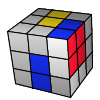R'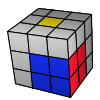In the example below the white sticker is pointing upwards. First we have to put the corner and edge pieces together, and put them where they need to be.R U2 R'U R U' R'We can never affect the solved blocks! In the situation below, instead of doing F U2 F' we are going to do U' R U2 R' so we don't mess up the blue-orange block.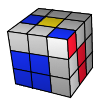F U2 F'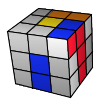U' R U2 R'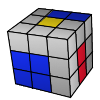To get faster sometimes we are going to use double turns. Just a reminder that the u letter in the algorithm means the rotation of the two upper layer clockwise. See full reference in the notation page.

## Be intuitive or learn algorithms?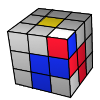An intuitive approach of this situation would suggest to solve this case in the following steps: to take the two pieces to the top layer, then joining them to insert where they belong to: (R U2 R') (F' U2 F) (U' R U R'). However the following algorithm is much quicker because it's the same series of movements repeating: (R U R' U') (R U R' U') (R U R' U')

Let's see the possible situtations you might meet in this stage. Cases grouped by the position of the white corner and the edge that needs to be attached to it:

### 1st: Easy cases

These are the lucky cases which can be solved in 3-4 moves.R U R'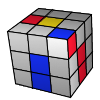F' U' F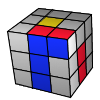U' F' U F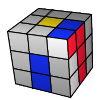U R U' R'

### 2nd case: Corner in bottom, edge in top layer

The first two should be familiar from the beginner's method: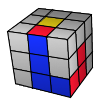(U R U' R') (U' F' U F)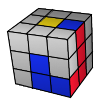(U' F' U F) (U R U' R')(F' U F) (U' F' U F)(R U R') (U' R U R')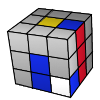(R U' R') (U R U' R')(F' U' F) (U F' U' F)

### 3rd case: Corner in top, edge in middle(R U R' U') (R U R' U') (R U R')(R U' R') (d R' U R)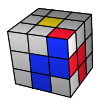(U F' U F) (U F' U2 F)(U F' U' F) (d' F U F')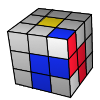(U' R U' R') (U' R U2 R')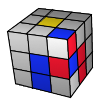(U' R U R') (d R' U' R)

### 4th case: Corner pointing outwards, edge in top layer

In this case we usually bring the cube to a basic case, reorienting the white corner in the first stage.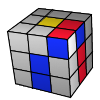(R U' R' U) (d R' U' R)(F' U F U') (d' F U F')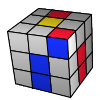(U F' U2 F) (U F' U2 F)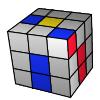(U' R U2 R') (U' R U2 R')(U F' U' F) (U F' U2 F)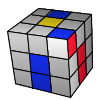(U' R U R') (U' R U2 R')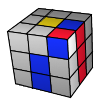(U' R U' R' U) (R U R')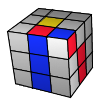(U F' U F U') (F' U' F)(U' R U R' U) (R U R')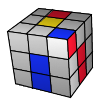(U F' U' F U') (F' U' F)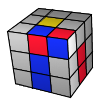(U F' U2 F U') (R U R')(U' R U2 R' U) (F' U' F)

### 5th case: Corner pointing upwards, edge in top layer(R U R' U') U' (R U R' U') (R U R')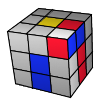y' (R' U' R U) U (R' U' R U) (R' U' R)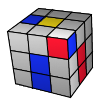(U2 R U R') (U R U' R')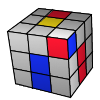(U2 F' U' F) (U' F' U F)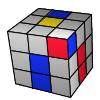(U R U2 R') (U R U' R')(U' F' U2 F) (U' F' U F)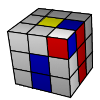(R U2 R') (U' R U R')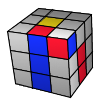(F' U2 F) (U F' U' F)

### 6th case: Corner in bottom, edge in middle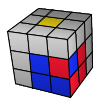(R U' R' d R' U2 R) (U R' U2 R)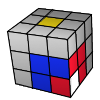(R U' R' U R U2 R') (U R U' R')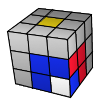(R U' R' U' R U R') (U' R U2 R')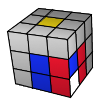(R U R' U' R U' R') (U d R' U' R)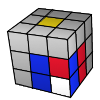(R U' R' d R' U' R) (U' R' U' R)